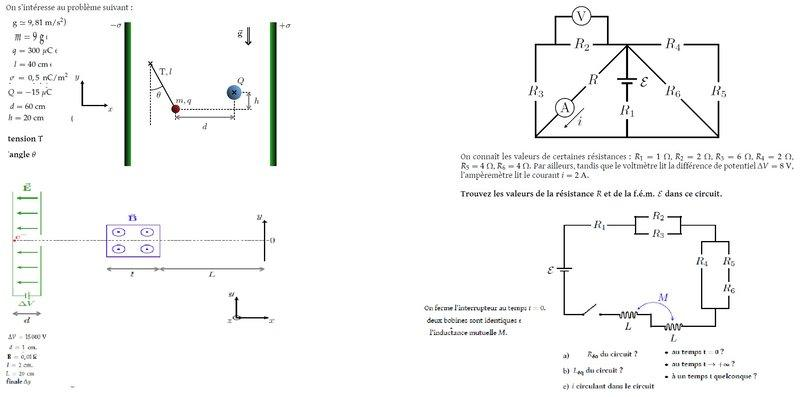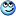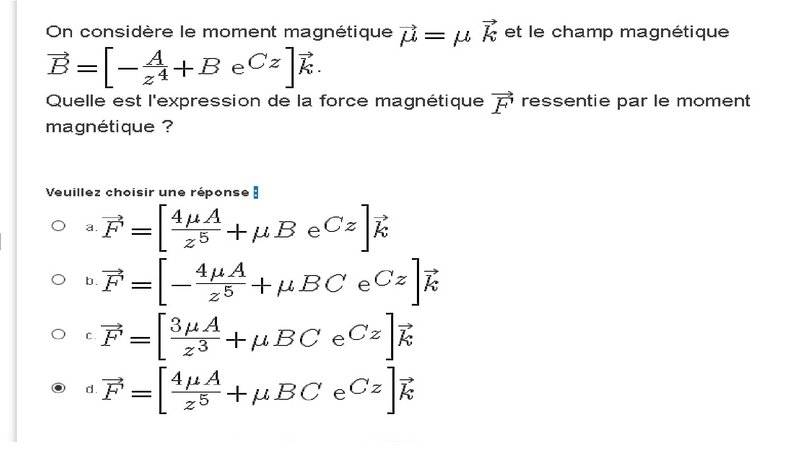# Radius of a particle's path in a B field and magentic momentum force

Member has been warned not to delete the template.

ok so 1- the magnetic momentum is = to u in the k axis ( xyz - ijk )
and the magnetic field B = -A/z4 + Be^Cz) , also in the k axis orientation

so the magnetic force F , that is applied on the magnetic momentum is given by

4 choices ; and as i can understnd it, 3 of them are the derivatives (2 bad ones ) of the b field and the other looks like the primitive of b . all 4 choice have u multiplied by it .

a- f = ( 4uA/z^5 + uBe^Cz) in k orientation
b- ( - 4uA/z^5 + uBCe^Cz) in k orientation
c- (3uA/z^3 + uBCe^Cz) in k orientation
d- (4uA/z^5 + uBCe^Cz) in k orientation

pretty sure the answer is D ,altough at first i thought it was b

as D is the correct derivtive of the b field, and its terms multiplied by the momentum value. So the magnetic momentum feels a force made by itself ?

again with a magnetic momentum of u = 1 (i) + 2 (k) , surrounded by a magnetic uniform field of b= 3 (i) + 4 (j) - 1 (k)
5rhe potential energy in mJ ?

is given by the dot product of u and b , correct? so the answer should be 11.4 , no ? or 539 ?

3 - 2 , 1 km wires ( so acting like infinite wires ) , both have same current , no direction specified , , separated by 1 meter distance and having between them a magnetic force of module 0.02 N . so the current value is 0.01???

the definition of 1 ampere has same conditions except the force between should be 2*10^-7 , so 2*10^-2

so 2*10^5 amps ???

last one of this thread ( couple of more threads coming up on different topics, been saving up almost a months worth of questions lol )

last one - charged particule reaches a uniform magnetic B field and velocity v , forming an a angle with the magnetic field . what is the expression of the relation , expressed as a ratio, of the radius and helical trajectory of the particule.

4 choices , all containing same term on the left side of the equation r/Ph=
a- 2pi/tan a
b- 1/2pi tan a
c- 2pi tan a
d- tan a / 2pi

so if particle , which is at rest, is subjected to a difference of potential of 15000 volts , over a 1cm distance, then enter the magnetic field b= . over a distance of 2cm. what will be its vertical displacement over 20cm .

gunning for d on this one, as the answer i ended after substitution and bit of algebra had cosine on the bottom .

although i cant figure out where they put the mass in that expression .

Please can anyone confirm my choice or say if theyre wrong? , i can justify my reasoning behind the choices , but each question is worded the exact same way which is the reason why im hesitating before submitting these answers .

thank you !#### Attachments

Last edited by a moderator:

BvU
Homework Helper
Hello thee,a) Looks to me as if you deleted the template altogether. Not a good idea at all.

1. Homework Statement

## The Attempt at a Solution

[/B]
been saving up almost a months worth of questions lol

b) Not a good idea either. perhaps you could have done the second or the third on your own after a little help with the first. Much better for your self-confidence and learning experience.

c) You can categorize, but we still want one exercise per thread -- for the benefit of others who are searching the forum

d) Use the superscript and subscript buttons (or ##\LaTeX## !) for legibility. And don't use bold face all over the place.

e) Can't really read the pictures -- have pity on us old and experienced lovers of physics who really want to help out !jtbell
Mentor
Not only is the text small and fuzzy, some of us here don't know French.lol, sweet , an active community .

first , greetings :)

second, my apologies , for not keeping the template , and for the small pictures.

i will not apologize for being french though.

still , i learn physics, you get to learn french, sounds like a good deal to me ;)

so , 1 thread per question , alrighty then , lets get started , (underscore for vector notation ?)

## Homework Statement

[/B]
the magnetic momentum is u = u k axis
and the magnetic field B =( -A/z4 + Be^Cz)

the magnetic force F , that is applied on the magnetic momentum is given

## Homework Equations

module of a vector
derivative of a function
= -u^B

## The Attempt at a Solution

4 choices ; and as i can understnd it, 3 of them are the derivatives (2 bad ones ) of the b field and the other looks like the primitive of b . all 4 choice have u multiplied by it .

a- f = ( 4uA/z^5 + uBe^Cz) in k orientation
b- ( - 4uA/z^5 + uBCe^Cz) in k orientation
c- (3uA/z^3 + uBCe^Cz) in k orientation
d- (4uA/z^5 + uBCe^Cz) in k orientation

pretty sure the answer is D ,as it is the correct derivative of the function given , or is it A cause of the minus sign of the vector product?

thank you! P.s the pictures are for other questions, after these 4 here , for which il make 3 separate threads .

BvU
Homework Helper
i will not apologize for being french though
No reason to do so. But in order to help as many people from all corners of the world, PF communicates in English. At the risk of triggering off-subject discussions: It's one of the most unsuitable and difficult candidates for a world language, but it's a de facto standard. So be it, regrettably.

still , I learn physics, you get to learn french, sounds like a good deal to meIn the same spirit: we're here to help, not to learn French -- I know much better ways to learn Frenchand sure done a lot of that. Also: if you aim for a career in physics, or in fact for almost any other academic pursuit, you'll need your English direly -- and yours can use a little brushing upso , 1 thread per question , alrighty then , lets get started
Let's.

## Homework Statement

[/B]
the magnetic momentum is u = u k axis
and the magnetic field B =( -A/z4 + Be^Cz)

the magnetic force F , that is applied on the magnetic momentum is given
1. What is magnetic momentum ?
Ik know of magnetic moment (and here) but that follows ##\vec \tau = \vec \mu \times \vec B## and ##\tau## is a torque, not a force...​

2. What is the correct expression for ##\vec B## ?
What you write is definitely not it:##\ \ ## z4 or z4 ? ##\ \ ## eCz or eCz ?​

3. You say: F is given, but you don't give it, so that means the answer should contain an F somewhere?

4. What is asked ?(And I don't mean A, B, C or D -- see below)

## Homework Equations

module of a vector
derivative of a function
= -u^B
I grant you the first one. However: in what way is it relevant ?

I also grant you the second one. I am prepared to assume you know how to differentiate a function. Very, very, very important in physics. But again: why is it relevant ?

Does the second one continue on the third line ? ... hmmmThe third one looks higly suspect to me.
I do not assume you mean -uB -- I really wouldn't know what to do with that -- but that you mean " = ##\vec \mu \times \vec B## " (you mention vector product later on) -- did you change notation halfway ? (Because in the problem statement there is also a "^", most likely with a different meaning).​

Where does the minus sign come from ?
What necessitates differentiation ?

## The Attempt at a Solution

What is intended here, is that you post your steps to come to the correct expression for whatever is being asked. Multiple choice is teacher laziness or computer stupidity and we shouldn't bow to that. So there !

Enough for today, this is going to be a long thread -- good thing we agreed to split up the problems: you can work in parallel !

Just so you know: I do not try to waste your time -- or mine. I am convinced you are very smart and eager to learn; and I am eager to help.

not a phd in physics no , lol , although i do like the subject , i would not be able to make a living out of it . aiming for chemistry :) :) :) , already got my 1st year undergrad and in biochemistry too . i need to get my average up, hence the time and effort i am putting in this .

here is all of what is given#### Attachments

and yes i do know how to differentiate . i do not intend to force my french on this marvelous community, and thank you for correcting my english, i am bilingual but some things dont translate as well.

and its an online course, and the only formula i found with than expression in the question is from a solenoid but as you can see, the question is laid out ambiguously

the only formula i know in that form is for current running through a solenoid , which is the one with z3 at denominator

the equation with the minus sign is the magnetic energy from a dipole in a magnetic field

the book recommended gives 2 formula for torque ( here we are in SI units so torque is in pounds if i am not mistaken )

force momentum is written as uB sine theta which can be expressed as u x B which would be the same as the force from electric momentum p x E

BvU
Homework Helper

(And your differentiation skills come in handy after all! But do check your expression ! )

I'm a physicist working in a chemical company based in NL -- "all" english. As we dutch say: mostly charcoal english (something you can only understand if you first translate the words back litterally -- par example: "your delicious wife")

BvU
Homework Helper
here we are in SI units so torque is in pounds if i am not mistaken
Oops

One of my teachers used to say the word for coal in french instead of carbon. Lol . Coal in french is charbon. but carbon in french is carbone lol. anyway il open a new thread for next question.

Ty!

BvU